Select Page
How Many Teaspoons in a Tablespoon? (With Conversion Chart!) | MUST READ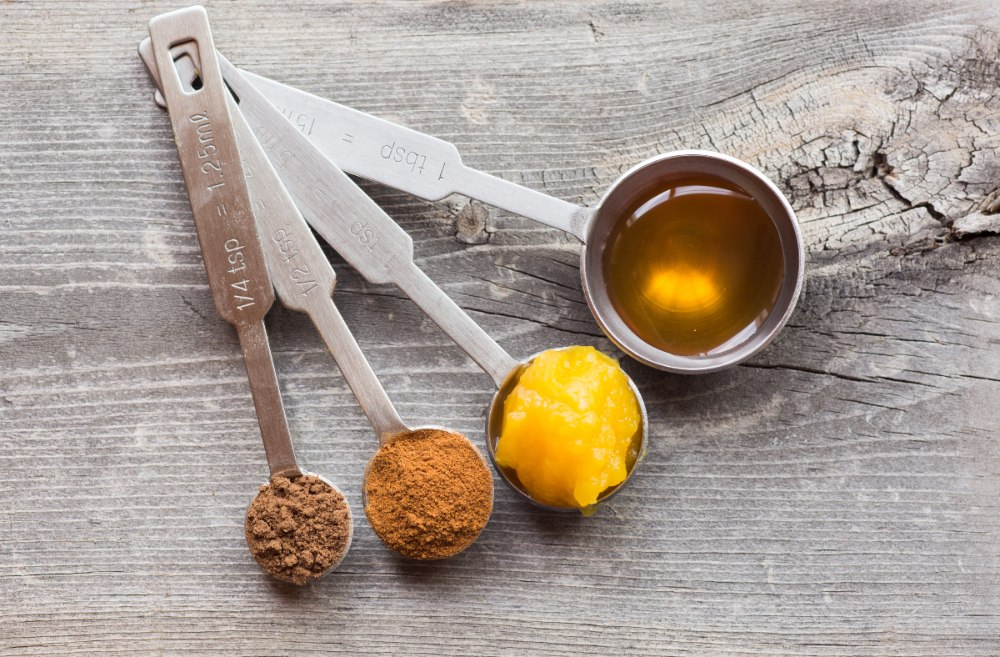Get Your Nord VPN account NOW ! claim the discount

In baking, one small inconsistency in the recipe could make or break a piece of bread or pastry. For instance, fluffy blueberry pancakes won’t be able to rise properly if you don’t use the right amount of baking powder. This is where measuring spoons will come in very handy.

But, what happens if you don’t have the right spoon size for the job? Practically speaking, this won’t be an issue if you know the basic conversions like how many teaspoons are in a tablespoon or vice versa.

So, continue reading if you want to learn how to convert these measurements properly. Aside from teaspoons to tablespoons, we’ve also listed below spoon equivalents of other basic kitchen measurement units like tablespoon to cup, ounces, grams, and even milliliters. This knowledge will also be very useful when you want to adjust the serving size of the dish you’re making. So read on to find the answer to all your questions!

## How Many Teaspoons in a Tablespoon?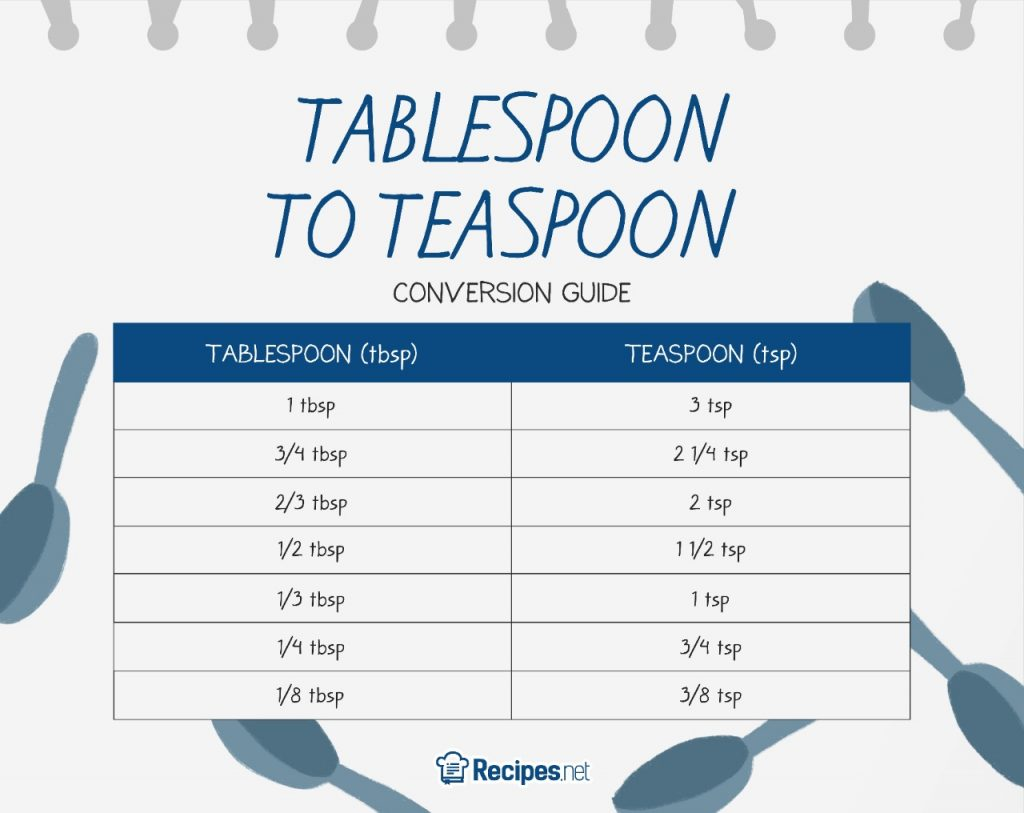For the likes of this fresh strawberry cheesecake, you can use the same spoon for measuring vanilla or lemon juice to minimize the number of dirty dishes you’d have to wash afterward. You can definitely achieve this even if their spoon units vary. So, how many teaspoons (tsp) are in a tablespoon (tbsp)?

To be exact, there are three teaspoons in a tablespoon. From there, you can adjust according to what a specific recipe calls for. Multiply the number if you have to scale up, or divide to scale down. Following the same logic, there are six half-teaspoons in a tablespoon. And, half a tablespoon equates to 1½ teaspoons.

You can refer to the measurement conversion chart above for the basic conversions. Simply take note that tbsp is the abbreviation for tablespoon, whereas tsp is for teaspoon. You can also use this table for converting the other way around (tablespoon to teaspoon).

## Imperial vs. Metric Measurements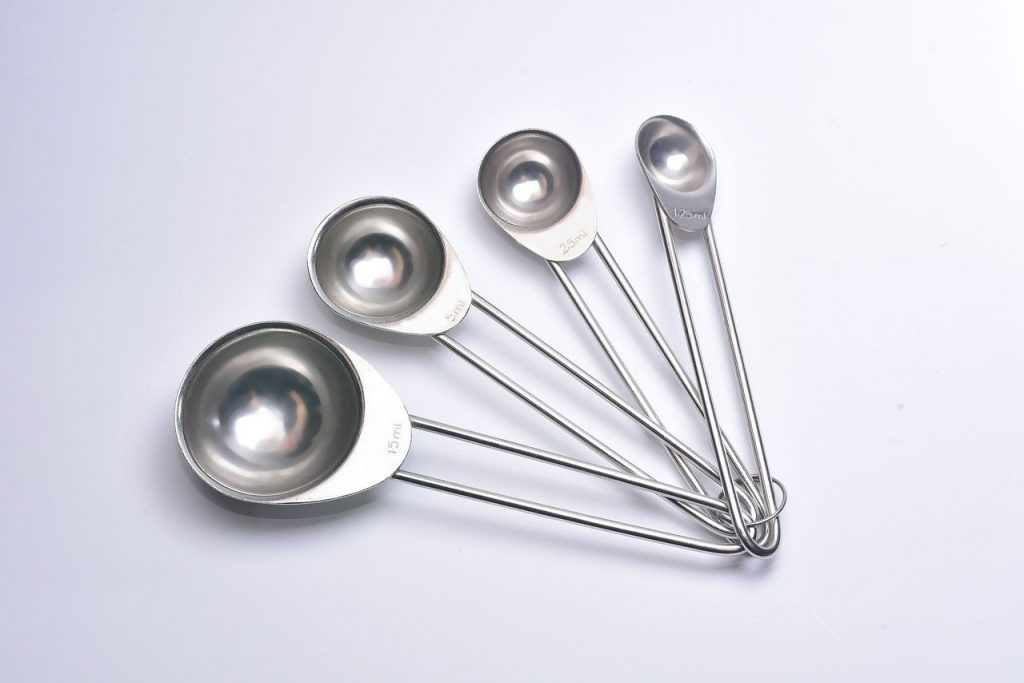Basically, there is a difference when it comes to the exact size of measuring spoons in different places. Countries such as the US, Myanmar, and Liberia all adhere to the imperial system. In that regard, a standard US tablespoon is equivalent to 0.5 fluid ounces or 14.79 ml and a teaspoon is 0.17 ounces or 4.93 ml.

On the other hand, the rest of the countries in the world use the metric system. There, a tablespoon is equal to 15 milliliters (ml) and a teaspoon is 5 milliliters or ml. This difference is very small, so it mostly doesn’t have a significant effect on most recipes. There is an exception, though. Although the metric system is employed in Australia, a tablespoon there is equal to 20 ml which may affect certain recipes. There is also a special dessert spoon often used in the UK which is equivalent to 10 ml.

Whether you adhere to the metric or imperial system, the conversion of teaspoons to tablespoons remains the same. So, you can easily achieve these heavenly souffle pancakes wherever you are in the world!

## More Handy Tablespoon Conversions to Know

Apart from knowing the conversion of how many teaspoons are in a tablespoon, you might also want to learn about how tablespoons compare to other basic measurements. Learn more about them below.

### How Many Tablespoons (tbsp) in a Cup? (And Vice Versa)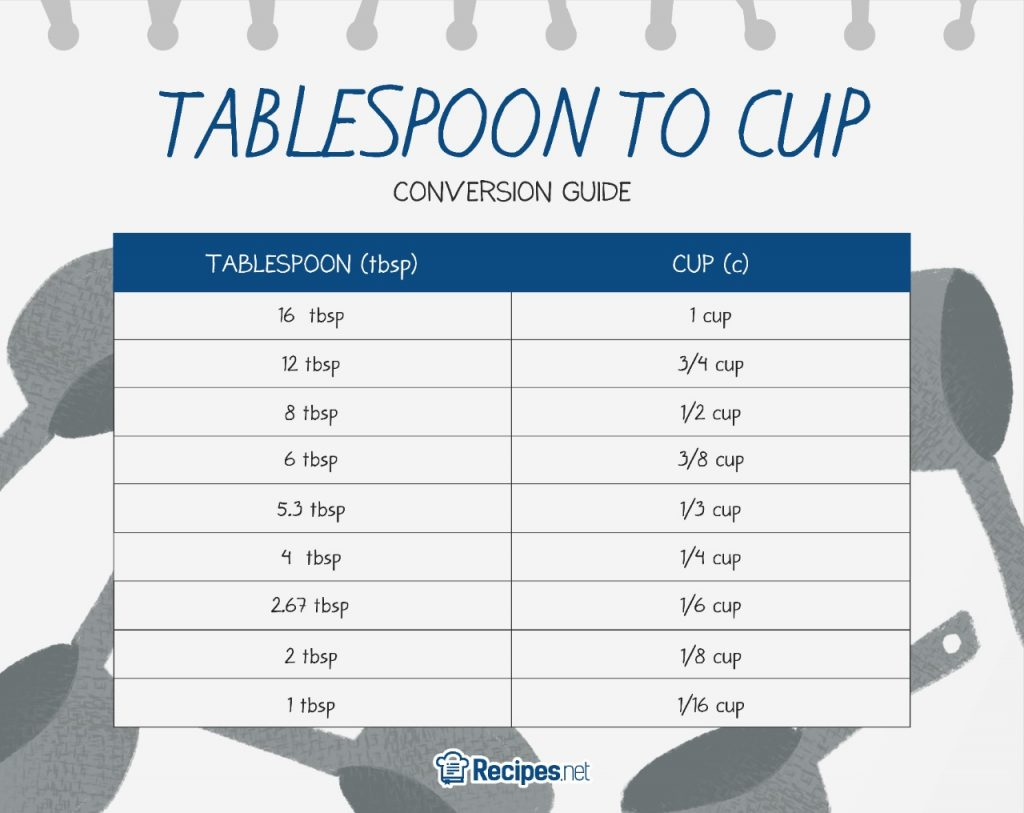Knowing the basic conversion of tablespoons to cups is very useful when you have to scale up or down ingredients for a recipe. For instance, when you want to make four batches of a wonderful no-bake chocolate cheesecake, you can use a measuring cup for the vanilla extract instead of manually adding them one by one.

As presented in the table above, there are 16 tablespoons in a cup. So it follows that ¾ cup to tbsp is equal to 12, and ½ cup to tbsp is eight. Meanwhile, ⅓ cup to tbsp is 5.3, ¼  cup to tbsp is four, ⅙ cup to tbsp is 2.67, ⅛ cup to tbsp is two, and so on. Meanwhile, there are 48 teaspoons in a cup.

In the same vein, six tablespoons are equivalent to ⅜ cups, eight tablespoons are ½, four tablespoons are ¼, and three tablespoons are 3/16.

### How Many Tablespoons (tbsp) in an Ounce (oz)?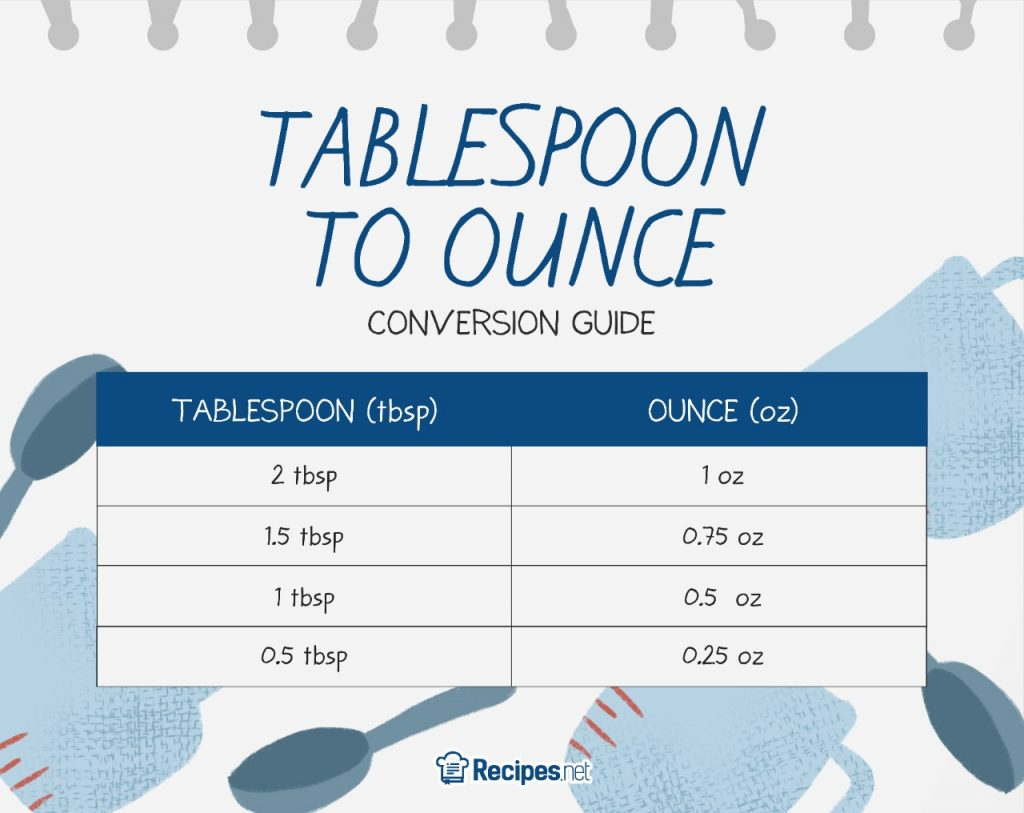For fluid measurements, knowing how to convert tablespoons to ounces will be very useful. Basically, there are two tablespoons in every ounce. So, how many ounces are there in a tablespoon? The answer is half an ounce.

In that sense, a fluid ounce is also equivalent to six teaspoons. And, one US teaspoon is about 0.17 ounces.

### How Many Grams (g) in a Tablespoon (tbsp)?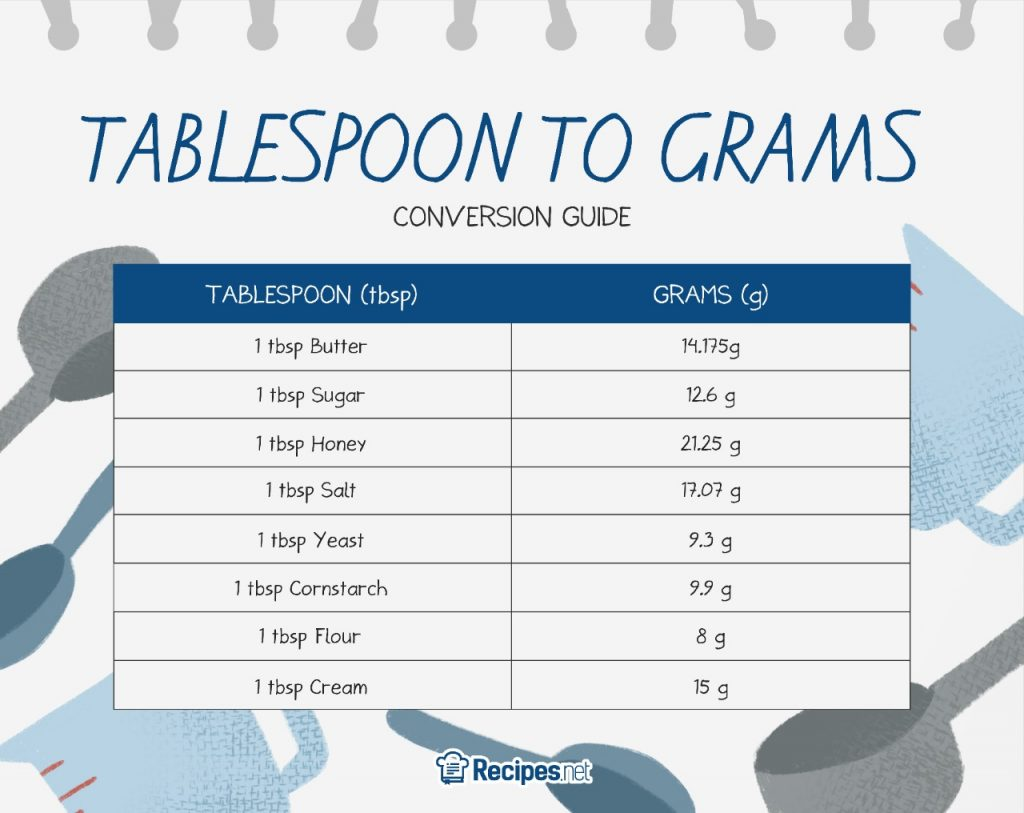If you want to know how many grams there are in a tablespoon, you can refer to the table above. It varies per ingredient, so we’ve gathered some of the most common baking ingredients for reference. You can also use the chart for converting tablespoons to grams!

### How Many Milliliters (ml) in a Tablespoon (tbsp)?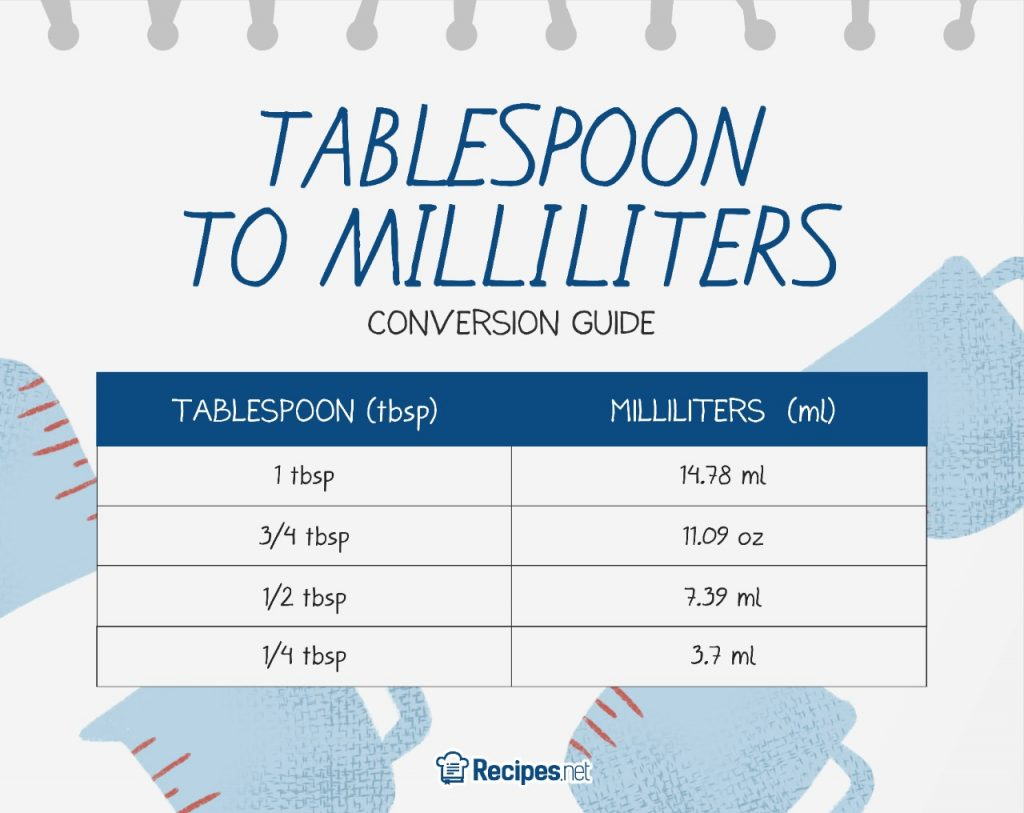Essentially, a tablespoon is equivalent to 14.78 ml, and one teaspoon is equal to 4.9 ml. Refer to the table above for the basic conversion.

## Tips to Remember Spoon Conversions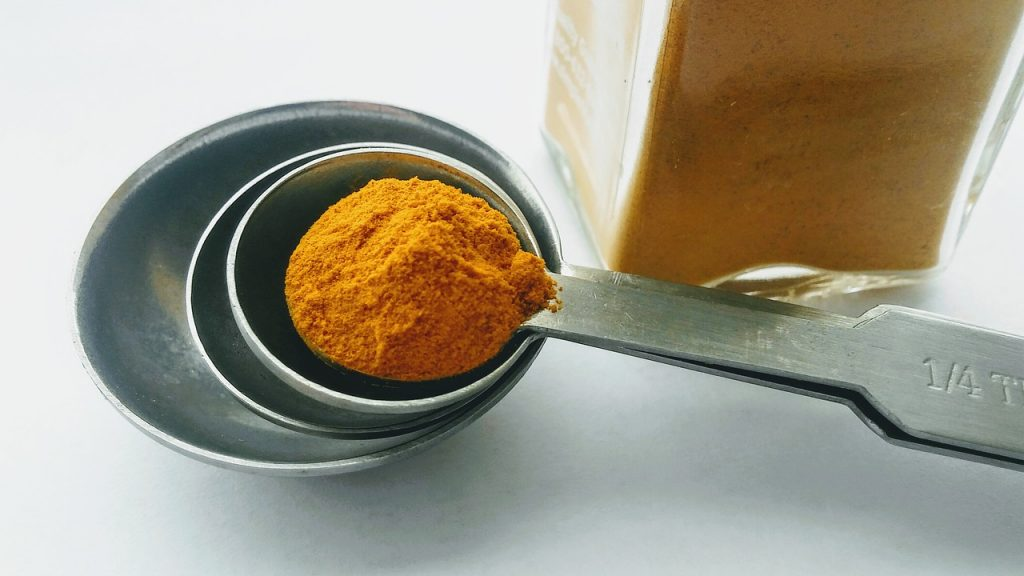Now that you’re all set with the basics, how do you remember how many teaspoons are in a tablespoon? To help you with this, we’ve put together simple tips that you can use as a reference whenever you have to face this dilemma. Find them below:

### Tablespoon (tbsp) to Teaspoon (tsp): Multiply by 3

Since there are three teaspoons in a tablespoon, you can simply multiply the number of tablespoons you need by three. With this in mind, you’ll have no problem figuring out answers to questions like, how many teaspoons does it take to make 1 and ½ tablespoons?

For a simpler representation, here is a quick formula:

Number of teaspoons = Number of tablespoons × 3

Here is an example using the problem mentioned above:

Number of teaspoons = 1 ½ × 3

Number of teaspoons = 4 ½

### Teaspoon (tsp) to Tablespoon (tbsp): Divide by 3

On the other hand, you can do the reverse if you have to figure out the number of tablespoons you need. Simply divide the number of teaspoons to three to find the answer.

Here is a quick formula for reference:

Number of tablespoons = Number of teaspoons ÷ 3